## Elliptic Plane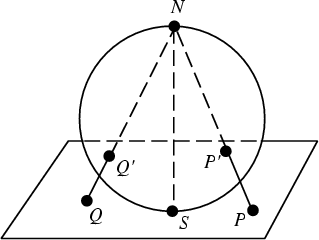The Real Projective Plane with elliptic Metric where the distance between two points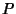and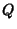is defined as the Radian Angle between the projection of the points on the surface of a Sphere (which is tangent to the plane at a point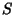) from the Antipode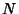of the tangent point.

References

Coxeter, H. S. M. Introduction to Geometry, 2nd ed. New York: Wiley, p. 94, 1969.